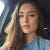# How to specify table size in MySQL database

It takes 2 actions to determine the size of tables in a database from a command line hosted on a MySQL or MariaDB server:

1. Connect to the database server with the command: mysql -u root -p (or simply mysql, if local authentication is not required).
2. Execute SQL query:

`SELECT`
`table_name AS `Table`,`
`round(((data_length + index_length) / 1024), 2) `Size in KB``
`FROM information_schema.TABLES`
`WHERE table_schema = "DBName";`

where, “DBName” is the name of the database, for which it is necessary to get the list of tables with sizes

To define the list and size of tables (in kilobytes) in the mysql database, you will need to perform a query:

`SELECT`
`table_name AS `Table`,`
`round(((data_length + index_length) / 1024 ), 2) `Size in KB``
`FROM information_schema.TABLES`
`WHERE table_schema = "mysql";`

`+---------------------------+------------+`
`| Table | Size in KB |`
`+---------------------------+------------+`
`| columns_priv | 4.00 |`
`| db | 13.94 |`
`| event | 2.00 |`
`| | 1.00 |`
`| general_log | 0.00 |`
`| help_category | 4.07 |`
`| help_keyword | 105.27 |`
`| help_relation | 28.04 |`
`| help_topic | 459.83 |`
`| host | 2.00 |`
`| ndb_binlog_index | 1.00 |`
`| plugin | 1.00 |`
`| proc | 2.00 |`
`| procs_priv | 4.00 |`
`| proxies_priv | 12.48 |`
`| servers | 1.00 |`
`| slow_log | 0.00 |`
`| tables_priv | 4.00 |`
`| time_zone | 1.00 |`
`| time_zone_leap_second | 1.00 |`
`| time_zone_name | 1.00 |`
`| time_zone_transition | 1.00 |`
`| time_zone_transition_type | 1.00 |`
`| user | 5.11 |`
`+---------------------------+------------+`
`24 rows in set (0.00 sec)`

To get the size of tables in Megabytes, you need a row:

`round(((data_length + index_length) / 1024 ), 2) `Size in KB``

substitute for

`round(((data_length + index_length) / 1024 / 1024), 2) `Size in MB'`

If the table list is very large, you can shorten the output by adding a condition to the WHERE design

`WHERE table_schema = "DBName"`
`AND table_name = "TableName";`

where, TableName is the name of the table for which you want to get the size

For example, to determine the size of the help_topic table in a mysql database, you will need to execute an SQL query:

`SELECT`
`table_name AS `Table`,`
`round(((data_length + index_length) / 1024 ), 2) `Size in KB``
`FROM information_schema.TABLES`
`WHERE table_schema = "mysql"`
`AND table_name = "help_topic"`

Result:

`+------------+------------+`
`| Table | Size in KB |`
`+------------+------------+`
`| help_topic | 459.83 |`
`+------------+------------+`
`1 row in set (0.00 sec)`

If it is necessary to obtain a limited list of tables, e.g. containing certain words in the title, you can shorten the output by adding a condition (Like “Filter”) to the WHERE construct.

`WHERE table_schema = "DBName"`
`AND table_name Like "Filter"`

For example, to determine the size of tables containing the word zone in the table name in a mysql database, you will need to execute an SQL query:

`SELECT`
`table_name AS `Table`,`
`round(((data_length + index_length) / 1024 ), 2) `Size in KB``
`FROM information_schema.TABLES`
`WHERE table_schema = "mysql"`
`AND table_name Like "%zone%"`

Result:
`+---------------------------+------------+`
`| Table | Size in KB |`
`+---------------------------+------------+`
`| time_zone | 1.00 |`
`| time_zone_leap_second | 1.00 |`
`| time_zone_name | 1.00 |`
`| time_zone_transition | 1.00 |`
`| time_zone_transition_type | 1.00 |`
`+---------------------------+------------+`
`5 rows in set (0.00 sec)`

PS The same information can be obtained using PHPMyAdmin, which provides a web interface for administering MySQL DBMS.

--

--